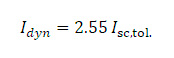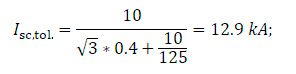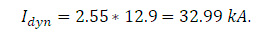# Calculation of short circuit currents

Isc,tol. = 0 kA
Idyn = 0 kA

Calculation of short circuit currents is carried out according to GOST 14794-79 (cl. 2.12.2-2.12.3), namely:

The permissible effective value of the periodic component of the short-circuit current  Isc,tol. kA, is determined by the formula:(when calculating Isc,tol. for a coupled reactor, X is substituted for X0.5 in the formula (1), and in cases of using a coupled reactor with series-connected lines, is substituted for Xc),

where U is the reactor voltage class, kV;

X is the nominal inductive resistance of a single reactor, Ohm;

X0.5 is the nominal inductive resistance of a coupled reactor (resistance of a line of the coupled reactor), Ohm;

Xc is the nominal inductive resistance of a coupled reactor, Ohm;

IRC is the rated current of the reactor, kA;

Inw – steady-state conditional short-circuit current in a network without the reactor in the place where the reactor shall be installed, at the rated network voltage corresponding to the reactor voltage class, kA. The Inw value shall be as follows: 125 kA for all reactors with a horizontal phase arrangement and for all reactors with a rated current equal to or greater than 1000 A, with a rated inductive resistance equal to or greater than 0.25 Ohms.

90 kA for reactors with vertical and stepwise phases with a rated current of less than 1000 A, with a rated inductive resistance equal to or greater than 0.40 Ohm.

70 kA for the remaining reactors.

The maximum instantaneous value of the current of electrodynamic resistance, in relation to which the calculations are performed and tests for electrodynamic resistance are carried out, is determined by the formula:where Idyn is the maximum instantaneous value of the electrodynamic resistance current for single reactors, as well as for coupled reactors with the current flowing in one line or in both lines in a consistent direction, kA.

EXAMPLE: Determination of short circuit currents for the RTST-10-1600-0.4 U3 reactor.

U = 10 kV;

Х = 0.4 Ohm;

Substitute the values in the formula for calculating the short-time thermal current:Substitute the obtained current value into the formula for calculating the dynamic resistance current (initial short-circuit current):